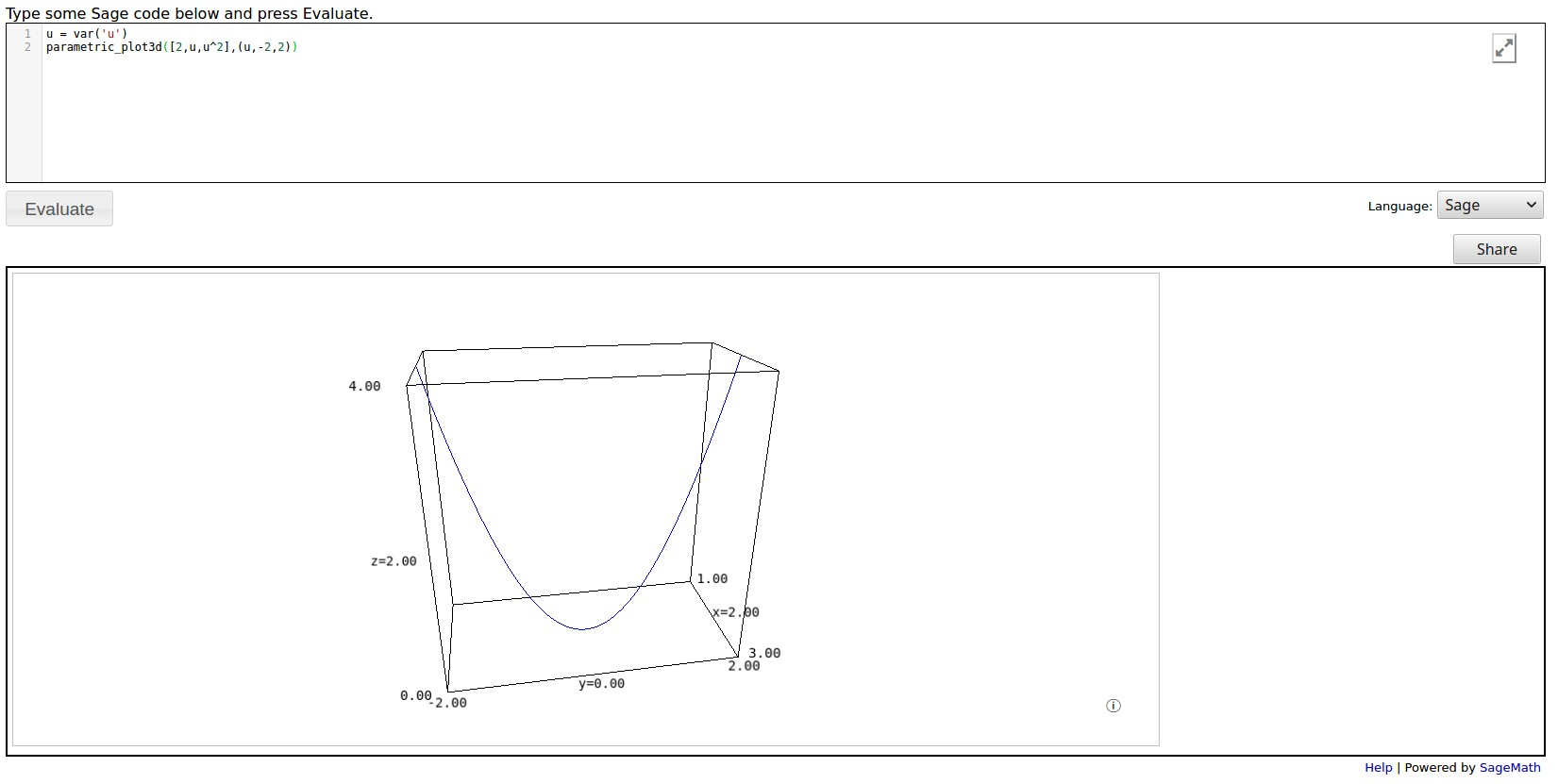# how do i plot a parabola in the x=2 plane?

I apologize, for I am very new to sage. Here is what I input; the error was that y was not defined.

f(x)=x^2
P=implicit_plot3d(lambda x,y,z:x-2,(-2,2),(-2,2),(-2,8))
plot(f)+plot3d(P)

edit retag close merge delete

You can use var('y') to define y as a variable, and similarly for z.

Sort by » oldest newest most voted

First of all, P is in itself a graphics object. Writing plot3d(P) does not make much sense.

Secondly, f is a one-variable function so that plot(f) is a 2 dimensional picture (that works). But then the addition plot(f)+plot3d(P) is trying to concatenate a 2-dimensional and a 3-dimensional picture that looks weird.

Now, to draw a curve in 3d you can for example use parametric_plot3d.

more

To get your plot using the parametric_plot3d command that vdelecroix suggested, you could try:

u = var('u')
parametric_plot3d([2,u,u^2],(u,-2,2))


The result, running in a SageCellServer is shown:more

Note that the command var('u') defines u: you should be able to use var('u') rather than u = var('u'). Unfortunately many of the examples in the Sage documentation needlessly use the longer version.